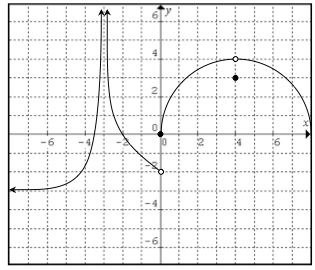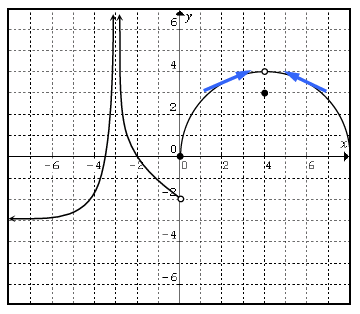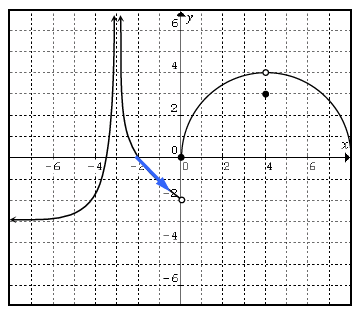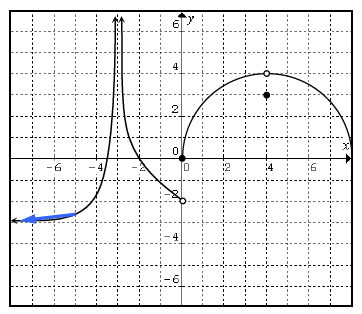### Home > PC > Chapter 7 > Lesson 7.3.1 > Problem7-98

7-98.

Use the graph at right to find the following limits.

1. $\lim\limits_ { x \rightarrow 4 } f ( x )$

Remember to approach on the curve from both sides of $x = 4$. What height is that?

Arrows added to right curve, left pointing up & right, left of open point (4, comma 4), & right pointing up & left, right of open point (4, comma 4).

2. $\lim\limits _ { x \rightarrow 0 ^ { - } } f ( x )$

Approach on the curve from the left (− side) of $x = 0$ only.
What height is that?

Arrow added to center curve, from the point (negative 2, comma 0), to open point (0, comma negative 2).

3. $\lim\limits _ { x \rightarrow - \infty } f ( x )$

As $x→-∞$, what height does the graph approach?

Arrow added to left curve, from approximately (negative 5, comma negative 3), down & left.

4. $\lim\limits _ { x \rightarrow 0 } f ( x )$

You must approach the same height from both sides for the limit to exist.

5. $\lim\limits _ { x \rightarrow - 3 } f ( x )$

Arrows added to left curve, left pointing up & right, left of dashed line, & right pointing up & left, right of dashed line.